It is currently Fri Jan 22, 2021 3:40 pm

 All times are UTC - 8 hours [ DST ]

 Print view Previous topic | Next topic
Author MessagePost subject: seki and dying: a fine line#1 Posted: Sun Feb 17, 2019 10:43 am
 Lives in gote

Posts: 635
Liked others: 58
Was liked: 116
So with Spring in the air and me having taken a trip down memory lane, I really wanted to play a game. I'm sick of being concerned with improving and whatnot. I decided to just play.
It's online, but I try not to blitz. Today it worked out okay at some points, though I completely ceased thinking and reading on the bottom, mindlessly imitating plays I remember from another context.

So while I got ahead initially, I lost my lead and was behind by 30 points there. Quite a lapse in concentration.
I do something desperate and it only takes two mistakes by my opponent to swing the game back to my favour. One is him missing a chance at seki instead of handing me the kill.

What follows is a great test (for someone like me, who is very prone to give away games where I'm leading, losing focus especially on computer Go) to stay focused and stay strong. Today I managed fine and black couldn't catch me with my pants down again.

Result:
Bad: I still played relatively blitz-y
Bad: the bottom sequence. I can't even ... Terrible ... Just noGood: I find my focus again to go for the kill and to fend off Black's further attacks, both on the left and in the right bottom corner.
Good: Attack with a plan and by gaining territory (a lot of it)

I want to take home one or two lessons from each game. So I hope to get some help deciding what lessons to learn from this one.
I think one is:
- don't push your opponent to your own weak group (I attacked to make profit, but in turn weakened my own group. In my defense, I thought it was alive with 2 eyes. It almost was, but in the end it almost died / sekied
Maybe for the 2nd lesson:
- Connect back up if you can instead of letting yourself getting surrounded without certainty of life.

What do you think? Good conclusions based on the game?

Thanks!

Some self review with Leela, comments in the file. Stops at move 164, except for a final comment at the end, about the corner up top.

TopPost subject: Re: seki and dying: a fine line#2 Posted: Mon Feb 18, 2019 2:01 pm
 TengenPosts: 5395
Location: Banbeck Vale
Liked others: 1027
Was liked: 1421
Rank: 1D AGA
GD Posts: 1512
Kaya handle: Test
`[go]\$\$ The F5 stone is too high. Undercut it while pincering.\$\$ ---------------------------------------\$\$ | . . . . X . . . . . . . . . . . . . . |\$\$ | . . . . . X O . . . . . . . . . . . . |\$\$ | . . X X X O . O . . . . . . . X . . . |\$\$ | X X O O O O . . . , . . . . . , . . . |\$\$ | . O . . . . . . . . . . . . . . X . . |\$\$ | . . . . . . . . . . . . . . . . . . . |\$\$ | . . . . . . . . . . . . . . . . . . . |\$\$ | . . . . . . . . . . . . . . . . . . . |\$\$ | . . . . . . . . . . . . . . . . . . . |\$\$ | . . . O . . . . . , . . . . . , . . . |\$\$ | . . . . . . . . . . . . . . . . . . . |\$\$ | . . . . . . . . . . . . . . . . . . . |\$\$ | . . . . . . . . . . . . . . . . . . . |\$\$ | . . O O . . . . . . . . . . . . . . . |\$\$ | . . X . . X . . . . . . . . . . . . . |\$\$ | . . . X . . . . . 2 . . . . . O . . . |\$\$ | . . . . . . . . . . . . . 1 . . . . . |\$\$ | . . . . . . . . . . . . . . . . . . . |\$\$ | . . . . . . . . . . . . . . . . . . . |\$\$ ---------------------------------------[/go]`

`[go]\$\$ Use that strength on the left\$\$ ---------------------------------------\$\$ | . . . . X . . . . . . . . . . . . . . |\$\$ | . . . . . X O . . . . . . . . . . . . |\$\$ | . . X X X O . O . . . O . . . X . . . |\$\$ | X X O O O O . . . 1 . . . . . , . . . |\$\$ | . O . . . . . . . . 2 . . . . . X . . |\$\$ | . . . . . . . . . . . . . . . . . . . |\$\$ | . . . . . . . . . . . . . . . . . . . |\$\$ | . . . . . . . . . . . . . . . . . . . |\$\$ | . . . . . . . . . . . . . . . . . . . |\$\$ | . . . O . . . . . , . . . . . , . . . |\$\$ | . . . . . . . . . . . . . . . . . . . |\$\$ | . . . . . . . . . . . . . . . . . . . |\$\$ | . . . . . . . . . . . . . . . . . . . |\$\$ | . . O O . . . . . . . . . . . . O . . |\$\$ | . . X . . X . . . . . . . . . . . . . |\$\$ | . . . X . . . . . , X . . . . O . . . |\$\$ | . . . . . . . . . . . . . X . . . . . |\$\$ | . . . . . . . . . . . . . . . . . . . |\$\$ | . . . . . . . . . . . . . . . . . . . |\$\$ ---------------------------------------[/go]`

`[go]\$\$ Position at move 35\$\$ ---------------------------------------\$\$ | . . . . X . . . . . . . . . . . . . . |\$\$ | . . . . . X O . . . . . . . . . . . . |\$\$ | . . X X X O . O . O O O 1 3 . X . . . |\$\$ | X X O O O O . . . X X X O . . , . . . |\$\$ | . O . . . . . . . . . . 2 . . . X . . |\$\$ | . . . . . . . . . . 4 . . . . . . . . |\$\$ | . . . . . . . . . . . . . . . . . . . |\$\$ | . . . . . . . . . . . . . . . . . . . |\$\$ | . . . . . . . . . . . . . . . . . . . |\$\$ | . . . O . . . . . , . . . . . , . . . |\$\$ | . . . . . . . . . . . . . . . . . . . |\$\$ | . . . . . . . . . . . . . . . . . . . |\$\$ | . . . . . . . . . . . . . . . . . . . |\$\$ | . . O O . . . . . . . . . . . . O . . |\$\$ | . . X . . X . . . . . . . . . . . . . |\$\$ | . . . X . . . . . , X . . . . O . . . |\$\$ | . . . . . . . . . . . . . X . . . . . |\$\$ | . . . . . . . . . . . . . . . . . . . |\$\$ | . . . . . . . . . . . . . . . . . . . |\$\$ ---------------------------------------[/go]`

`[go]\$\$ This is the vital point\$\$ ---------------------------------------\$\$ | . . . . X . . . . . . . . . . . . . . |\$\$ | . . . . . X O . . . . . . . . . . . . |\$\$ | . . X X X O . O . O O O X X X X . . . |\$\$ | X X O O O O . . . X X X O O O , . . . |\$\$ | . O . . . . . . . . . . . . . . X . . |\$\$ | . . . . . . . . . . . . . . . . . . . |\$\$ | . . . . . . . . . . . X . O . O X . . |\$\$ | . . . . . . . . . . . . . . O X O . . |\$\$ | . . . . . . . . . . . . . O . 1 . . . |\$\$ | . . . O . . . . . , . . . O X X X 2 . |\$\$ | . . . . . . . . . . . . . X O O O . . |\$\$ | . . . . . . . . . . . . . . X X O . . |\$\$ | . . . . . . . . . . . . . . . . . . . |\$\$ | . . O O . . . . . . . . . . . . O . . |\$\$ | . . X . . X . . . . . . . . . . . . . |\$\$ | . . . X . . . . . , X . . . . O . . . |\$\$ | . . . . . . . . . . . . . X . . . . . |\$\$ | . . . . . . . . . . . . . . . . . . . |\$\$ | . . . . . . . . . . . . . . . . . . . |\$\$ ---------------------------------------[/go]`

`[go]\$\$ So big that it is worth repeating: play here\$\$ ---------------------------------------\$\$ | . . . . X . . . . . . . . . . . . . . |\$\$ | . . . . . X O . . . . . . . . . . . . |\$\$ | . . X X X O . O . O O O X X X X . . . |\$\$ | X X O O O O . . . X X X O O O , 1 . . |\$\$ | . O . . . . . . . . . . . . . O X . . |\$\$ | . . . . . . . . . . . . . . . O O X . |\$\$ | . . . . . . . . . . . X . O . O X X . |\$\$ | . . . . . . . . . . . . . . O X O . . |\$\$ | . . . . . . . . . . . . . O . X . . . |\$\$ | . . . O . . . . . , . . . O X X X 2 . |\$\$ | . . . . . . . . . . . . . X O O O . . |\$\$ | . . . . . . . . . . . . . . X X O . . |\$\$ | . . . . . . . . . . . . . . . . . . . |\$\$ | . . O O . . . . . . . . . . . . O . . |\$\$ | . . X . . X . . . . . . . . . . . . . |\$\$ | . . . X . . . . . , X . . . . O . . . |\$\$ | . . . . . . . . . . . . . X . . . . . |\$\$ | . . . . . . . . . . . . . . . . . . . |\$\$ | . . . . . . . . . . . . . . . . . . . |\$\$ ---------------------------------------[/go]`

`[go]\$\$W If you want to attack, get the vital point, and force him toward your strength\$\$ ---------------------------------------\$\$ | . . . . X . . . . . . . . . . . . . . |\$\$ | . . . . . X O . . . . . . . . . . . . |\$\$ | . . X X X O . O . O O O X X X X . . . |\$\$ | X X O O O O . . . X X X O O O , X . . |\$\$ | . O . . . . . . . . . . . . . O X . . |\$\$ | . . . . . . . . . . 1 . . . . O O X . |\$\$ | . . . . . . . . . . . X . O . O X X . |\$\$ | . . . . . . . . . . . . . . O X O . . |\$\$ | . . . . . . . . . . . . . O . X . . . |\$\$ | . . . O . . . . . , . O . O X X X X . |\$\$ | . . . . . . . . . . . . O X O O O . . |\$\$ | . . . . . . . . . . . . O X X X O . . |\$\$ | . . . . . . . . . . . O X X . . . . . |\$\$ | . . O O . . . . . . . . O X . . O . . |\$\$ | . . X . . X . . . . . . . . . . . . . |\$\$ | . . . X . . . . . , X . . . . O . . . |\$\$ | . . . . . . . . . . . . . X . . . . . |\$\$ | . . . . . . . . . . . . . . . . . . . |\$\$ | . . . . . . . . . . . . . . . . . . . |\$\$ ---------------------------------------[/go]`

`[go]\$\$W However, this may be bigger.\$\$ ---------------------------------------\$\$ | . . . . X . . . . . . . . . . . . . . |\$\$ | . . . . . X O . . . . . . . . . . . . |\$\$ | . . X X X O . O . O O O X X X X . . . |\$\$ | X X O O O O . . . X X X O O O , X . . |\$\$ | . O . . . . . . . . . . . . . O X . . |\$\$ | . . . . . . . . . . . . . . . O O X . |\$\$ | . . . . . . . . . . . X . O . O X X . |\$\$ | . . . . . . . . . . . . . . O X O . . |\$\$ | . . . . . . . . . . . . . O . X . . . |\$\$ | . . . O . . . . . , . O . O X X X X . |\$\$ | . . . . . . . . . . . . O X O O O . . |\$\$ | . . . . . . . . . . . . O X X X O . . |\$\$ | . . . . . . . . . . . O X X . . . . . |\$\$ | . . O O . . . . . . . . O X . . O . . |\$\$ | . . X . . X . . . . . . . 1 . . . . . |\$\$ | . . . X . . . . . , X . . . . O . . . |\$\$ | . . . . . . . . . . . . . X . . . . . |\$\$ | . . . . . . . . . . . . . . . . . . . |\$\$ | . . . . . . . . . . . . . . . . . . . |\$\$ ---------------------------------------[/go]`

Note in the fourth and seventh diagrams, I suggest plays that probably won't kill, but will saddle your opponent with weak groups. Sometimes the burden of continually defending those groups may be worse than losing them.

Also, check https://senseis.xmp.net/?VitalPoint. Try to grasp the essence of the concept of a vital point.

TopPost subject: Re: seki and dying: a fine line#3 Posted: Mon Feb 18, 2019 4:05 pm
 GoseiPosts: 1761
Location: Ghent, Belgium
Liked others: 269
Was liked: 811
Rank: KGS 2d OGS 1d Fox 4d
KGS: Artevelde
OGS: Knotwilg
Online playing schedule: UTC 18:00 - 22:00
At 237 what if Black D7? I had to look at it a few times.

TopPost subject: Re: seki and dying: a fine line#4 Posted: Mon Feb 18, 2019 7:07 pm
 TengenPosts: 5395
Location: Banbeck Vale
Liked others: 1027
Was liked: 1421
Rank: 1D AGA
GD Posts: 1512
Kaya handle: Test
Knotwilg wrote:
At 237 what if Black D7? I had to look at it a few times.

It is tricky. I think A10 defends.

TopPost subject: Re: seki and dying: a fine line#5 Posted: Mon Feb 18, 2019 10:56 pm
 Honinbo

Posts: 10625
Liked others: 3558
Was liked: 3329
Joaz Banbeck wrote:
Knotwilg wrote:
At 237 what if Black D7? I had to look at it a few times.

It is tricky. I think A10 defends.

Then White can run out, right?_________________
At some point, doesn't thinking have to go on?

My two main guides in life:
My mother and my wife.Everything with love. Stay safe.

 This post by Bill Spight was liked by: Joaz Banbeck
TopPost subject: Re: seki and dying: a fine line#6 Posted: Mon Feb 18, 2019 11:01 pm
 Honinbo

Posts: 10625
Liked others: 3558
Was liked: 3329
Several comments with some tricky variations. No guarantee I got everything right, OC.Main foci:

1) As a rule, do not attack from behind. Get ahead or in front.

2) Don't go after the small stuff. Not at first, anyway.

3) Attack potential eyes, as a rule.

4) Keima for attack.

_________________
At some point, doesn't thinking have to go on?

My two main guides in life:
My mother and my wife.Everything with love. Stay safe.

Last edited by Bill Spight on Tue Feb 19, 2019 1:03 am, edited 3 times in total.
 This post by Bill Spight was liked by: Joaz Banbeck
TopPost subject: Re: seki and dying: a fine line#7 Posted: Mon Feb 18, 2019 11:50 pm
 TengenPosts: 5395
Location: Banbeck Vale
Liked others: 1027
Was liked: 1421
Rank: 1D AGA
GD Posts: 1512
Kaya handle: Test
Joaz Banbeck wrote:
Knotwilg wrote:
At 237 what if Black D7? I had to look at it a few times.

It is tricky. I think A10 defends.

Although with black's best play, white has to give up A5 and B5, but he escapes northward.

`[go]\$\$ Position at move 237\$\$ ---------------------------------------\$\$ | . . . . X . . . . . . . . . . . . . . |\$\$ | . X . . . X O . . . . O O X . . . . . |\$\$ | . . X X X O . O . O O O X X X X . . . |\$\$ | X X O O O O . . O X X X O O O X X . . |\$\$ | O O . . . . . . . O . X X O O O X . . |\$\$ | . . . O . . . O O X X X X X . O O X . |\$\$ | . . O O O X X O X X O X O O O O X X . |\$\$ | . 8 O . O O X O X . O X X X O X O . . |\$\$ | 6 X X O X O O X X X O O X O O X . . . |\$\$ | 2 O X , X X O X O X X O O O X X X X . |\$\$ | . O X X . O . O O X O . O X O O O O X |\$\$ | 3 O O X X O O O X O O O O X X X O X X |\$\$ | 5 O 4 1 O X X X X X X O X X . . O X . |\$\$ | . 7 O O O O O O X X . X . X . . O O . |\$\$ | O O X X O X X X . X . X . X O O . . . |\$\$ | X X . X X . . O . , X . X X X O . O O |\$\$ | . . . . . . X . . X . . . X X O O X O |\$\$ | . . . . . . . O . . X . X X O O X . X |\$\$ | . . . . . . . . . . . X X O . O . X . |\$\$ ---------------------------------------[/go]`

Trying to save those two stones is not good for white.

`[go]\$\$\$\$ ---------------------------------------\$\$ | . . . . X . . . . . . . . . . . . . . |\$\$ | . X . . . X O . . . . O O X . . . . . |\$\$ | . . X X X O . O . O O O X X X X . . . |\$\$ | X X O O O O . . O X X X O O O X X . . |\$\$ | O O . . . . . . . O . X X O O O X . . |\$\$ | . . . O . . . O O X X X X X . O O X . |\$\$ | . . O O O X X O X X O X O O O O X X . |\$\$ | 9 . O . O O X O X . O X X X O X O . . |\$\$ | 8 X X O X O O X X X O O X O O X . . . |\$\$ | 2 O X , X X O X O X X O O O X X X X . |\$\$ | . O X X . O . O O X O . O X O O O O X |\$\$ | 3 O O X X O O O X O O O O X X X O X X |\$\$ | 4 O 6 1 O X X X X X X O X X . . O X . |\$\$ | 7 5 O O O O O O X X . X . X . . O O . |\$\$ | O O X X O X X X . X . X . X O O . . . |\$\$ | X X . X X . . O . , X . X X X O . O O |\$\$ | . . . . . . X . . X . . . X X O O X O |\$\$ | . . . . . . . O . . X . X X O O X . X |\$\$ | . . . . . . . . . . . X X O . O . X . |\$\$ ---------------------------------------[/go]`

How many of us would see A8 over the board?

TopPost subject: Re: seki and dying: a fine line#8 Posted: Tue Feb 19, 2019 12:09 am
 Honinbo

Posts: 10625
Liked others: 3558
Was liked: 3329
`[go]\$\$ Position at move 237\$\$ ---------------------------------------\$\$ | . . . . X . . . . . . . . . . . . . . |\$\$ | . X . . . X O . . . . O O X . . . . . |\$\$ | . . X X X O . O . O O O X X X X . . . |\$\$ | X X O O O O . . O X X X O O O X X . . |\$\$ | O O . . . . . . . O . X X O O O X . . |\$\$ | . . . O . . . O O X X X X X . O O X . |\$\$ | . . O O O X X O X X O X O O O O X X . |\$\$ | . . O . O O X O X . O X X X O X O . . |\$\$ | 6 X X O X O O X X X O O X O O X . . . |\$\$ | 2 O X , X X O X O X X O O O X X X X . |\$\$ | . O X X . O . O O X O . O X O O O O X |\$\$ | 3 O O X X O O O X O O O O X X X O X X |\$\$ | 5 O . 1 O X X X X X X O X X . . O X . |\$\$ | . 4 O O O O O O X X . X . X . . O O . |\$\$ | O O X X O X X X . X . X . X O O . . . |\$\$ | X X . X X . . O . , X . X X X O . O O |\$\$ | . . . . . . X . . X . . . X X O O X O |\$\$ | . . . . . . . O . . X . X X O O X . X |\$\$ | . . . . . . . . . . . X X O . O . X . |\$\$ ---------------------------------------[/go]`

`[go]\$\$ Position at move 237\$\$ ---------------------------------------\$\$ | . . . . X . . . . . . . . . . . . . . |\$\$ | . X . . . X O . . . . O O X . . . . . |\$\$ | . . X X X O . O . O O O X X X X . . . |\$\$ | X X O O O O . . O X X X O O O X X . . |\$\$ | O O . . . . . . . O . X X O O O X . . |\$\$ | . 9 . O . . . O O X X X X X . O O X . |\$\$ | . . O O O X X O X X O X O O O O X X . |\$\$ | . . O . O O X O X . O X X X O X O . . |\$\$ | 8 X X O X O O X X X O O X O O X . . . |\$\$ | 2 O X , X X O X O X X O O O X X X X . |\$\$ | . O X X . O . O O X O . O X O O O O X |\$\$ | 7 O O X X O O O X O O O O X X X O X X |\$\$ | . O 5 1 O X X X X X X O X X . . O X . |\$\$ | 4 3 O O O O O O X X . X . X . . O O . |\$\$ | O O X X O X X X . X . X . X O O . . . |\$\$ | X X . X X . . O . , X . X X X O . O O |\$\$ | . . . . . . X . . X . . . X X O O X O |\$\$ | . . . . . . . O . . X . X X O O X . X |\$\$ | . . . . . . . . . . . X X O . O . X . |\$\$ ---------------------------------------[/go]`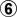@ 3.

Edit: Oh!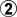doesn't work, does it? White has to lose some stones.

_________________
At some point, doesn't thinking have to go on?

My two main guides in life:
My mother and my wife.Everything with love. Stay safe.

TopPost subject: Re: seki and dying: a fine line#9 Posted: Tue Feb 19, 2019 1:55 am
 Honinbo

Posts: 10625
Liked others: 3558
Was liked: 3329
`[go]\$\$c Seki\$\$ ---------------------------------------\$\$ | . . . . X . . . . . . . . . . . . . . |\$\$ | . X . . . X O . . . . O O X . . . . . |\$\$ | . . X X X O . O . O O O X X X X . . . |\$\$ | X X O O O O . . O X X X O O O X X . . |\$\$ | O O . . . . . . . O . X X O O O X . . |\$\$ | . . . O . . . O O X X X X X . O O X . |\$\$ | . . O O O X X O X X O X O O O O X X . |\$\$ | 4 6 O 8 O O X O X . O X X X O X O . . |\$\$ | 5 X X O X O O X X X O O X O O X . . . |\$\$ | 3 O X 0 X X O X O X X O O O X X X X . |\$\$ | 7 O X X b O . O O X O . O X O O O O X |\$\$ | 9 O O X X O O O X O O O O X X X O X X |\$\$ | a O . 1 O X X X X X X O X X . . O X . |\$\$ | . 2 O O O O O O X X . X . X . . O O . |\$\$ | O O X X O X X X . X . X . X O O . . . |\$\$ | X X . X X . . O . , X . X X X O . O O |\$\$ | . . . . . . X . . X . . . X X O O X O |\$\$ | . . . . . . . O . . X . X X O O X . X |\$\$ | . . . . . . . . . . . X X O . O . X . |\$\$ ---------------------------------------[/go]`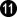@ a ,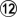@ b

`[go]\$\$c Ko\$\$ ---------------------------------------\$\$ | . . . . X . . . . . . . . . . . . . . |\$\$ | . X . . . X O . . . . O O X . . . . . |\$\$ | . . X X X O . O . O O O X X X X . . . |\$\$ | X X O O O O . . O X X X O O O X X . . |\$\$ | O O . . . . . . . O . X X O O O X . . |\$\$ | . . . O . . . O O X X X X X . O O X . |\$\$ | . 8 O O O X X O X X O X O O O O X X . |\$\$ | 9 7 O 0 O O X O X . O X X X O X O . . |\$\$ | 6 X X O X O O X X X O O X O O X . . . |\$\$ | 3 O X , X X O X O X X O O O X X X X . |\$\$ | 4 O X X . O . O O X O . O X O O O O X |\$\$ | . O O X X O O O X O O O O X X X O X X |\$\$ | 5 O . 1 O X X X X X X O X X . . O X . |\$\$ | . 2 O O O O O O X X . X . X . . O O . |\$\$ | O O X X O X X X . X . X . X O O . . . |\$\$ | X X . X X . . O . , X . X X X O . O O |\$\$ | . . . . . . X . . X . . . X X O O X O |\$\$ | . . . . . . . O . . X . X X O O X . X |\$\$ | . . . . . . . . . . . X X O . O . X . |\$\$ ---------------------------------------[/go]`

`[go]\$\$cm11 Ko\$\$ ---------------------------------------\$\$ | . . . . X . . . . . . . . . . . . . . |\$\$ | . X . . . X O . . . . O O X . . . . . |\$\$ | . . X X X O . O . O O O X X X X . . . |\$\$ | X X O O O O . . O X X X O O O X X . . |\$\$ | O O . . . . . . . O . X X O O O X . . |\$\$ | . . . O . . . O O X X X X X . O O X . |\$\$ | . O O O O X X O X X O X O O O O X X . |\$\$ | X X O O O O X O X . O X X X O X O . . |\$\$ | O X X O X O O X X X O O X O O X . . . |\$\$ | 5 O X 2 X X O X O X X O O O X X X X . |\$\$ | O O X X 4 O . O O X O . O X O O O O X |\$\$ | 6 O O X X O O O X O O O O X X X O X X |\$\$ | X O 1 X O X X X X X X O X X . . O X . |\$\$ | 3 O O O O O O O X X . X . X . . O O . |\$\$ | O O X X O X X X . X . X . X O O . . . |\$\$ | X X . X X . . O . , X . X X X O . O O |\$\$ | . . . . . . X . . X . . . X X O O X O |\$\$ | . . . . . . . O . . X . X X O O X . X |\$\$ | . . . . . . . . . . . X X O . O . X . |\$\$ ---------------------------------------[/go]`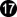throw in at 13,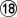= ko

Where is a good ko threat for Black?G-19, huh?

_________________
At some point, doesn't thinking have to go on?

My two main guides in life:
My mother and my wife.Everything with love. Stay safe.

TopPost subject: Re: seki and dying: a fine line#10 Posted: Tue Feb 19, 2019 12:27 pm
 Honinbo

Posts: 10625
Liked others: 3558
Was liked: 3329
And the root of the problem,, seems to be move 204.

`[go]\$\$W Move 204\$\$ ---------------------------------------\$\$ | . . . . X . . . . . . . . . . . . . . |\$\$ | . X . . . X O . . . . . . . . . . . . |\$\$ | . . X X X O . O . O O O X X X X . . . |\$\$ | X X O O O O . . O X X X O O O X X . . |\$\$ | O O . . . . . . . O . X X O O O X . . |\$\$ | . . . O . . . O O X X X X X . O O X . |\$\$ | . . O O O X X O X X O X O O O O X X . |\$\$ | . . O . O O X O X . O X X X O X O . . |\$\$ | . X X O X O O X X X O O X O O X . . . |\$\$ | . O X , X X O X O X X O O O X X X X . |\$\$ | . O X X . O . O O X O . O X O O O . . |\$\$ | . O O X X O O O X O O O O X X X O . . |\$\$ | . 1 . . O X X X X X X O X X . . . . . |\$\$ | b a O O O O O O X X . X O X . . O . . |\$\$ | O O X c O X X X . X . X O . . . . . . |\$\$ | X X . X X . . O . , X . X X . O . . . |\$\$ | . . . . . . X . . X . . . X . . . . . |\$\$ | . . . . . . . O . . X . X . . . . . . |\$\$ | . . . . . . . . . . . X . . . . . . . |\$\$ ---------------------------------------[/go]`

At first glance,appears to live, with a one space eye at "a" or "b" without having to make another play. But Knotwilg shows us that that is not so after Black fills the dame at "c".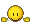`[go]\$\$W Prevents liberty stealing throw-in\$\$ ---------------------------------------\$\$ | . . . . X . . . . . . . . . . . . . . |\$\$ | . X . . . X O . . . . . . . . . . . . |\$\$ | . . X X X O . O . O O O X X X X . . . |\$\$ | X X O O O O . . O X X X O O O X X . . |\$\$ | O O b . . . . . . O . X X O O O X . . |\$\$ | . a . O . . . O O X X X X X . O O X . |\$\$ | . . O O O X X O X X O X O O O O X X . |\$\$ | . . O . O O X O X . O X X X O X O . . |\$\$ | . X X O X O O X X X O O X O O X . . . |\$\$ | 3 O X , X X O X O X X O O O X X X X . |\$\$ | . O X X . O . O O X O . O X O O O . . |\$\$ | 4 O O X X O O O X O O O O X X X O . . |\$\$ | 2 c 5 . O X X X X X X O X X . . . . . |\$\$ | . 1 O O O O O O X X . X O X . . O . . |\$\$ | O O X B O X X X . X . X O . . . . . . |\$\$ | X X . X X . . O . , X . X X . O . . . |\$\$ | . . . . . . X . . X . . . X . . . . . |\$\$ | . . . . . . . O . . X . X . . . . . . |\$\$ | . . . . . . . . . . . X . . . . . . . |\$\$ ---------------------------------------[/go]`

The solid connection,in this diagram, does not make two eyes, either, but it prevents the liberty stealing throw-in at that point.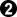-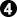holds White to one eye, but it is a big eye. Even with the dame at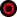filled, the big eye gives ample liberties for White to win the semeai. White can answer "a" at "b". Note that if Black triesat 5, White "c" makes two eyes.

`[go]\$\$W Easy life\$\$ ---------------------------------------\$\$ | . . . . X . . . . . . . . . . . . . . |\$\$ | . X . . . X O . . . . . . . . . . . . |\$\$ | . . X X X O . O . O O O X X X X . . . |\$\$ | X X O O O O . . O X X X O O O X X . . |\$\$ | O O . . . . . . . O . X X O O O X . . |\$\$ | . . . O . . . O O X X X X X . O O X . |\$\$ | . . O O O X X O X X O X O O O O X X . |\$\$ | . . O . O O X O X . O X X X O X O . . |\$\$ | . X X O X O O X X X O O X O O X . . . |\$\$ | 6 O X , X X O X O X X O O O X X X X . |\$\$ | 7 O X X . O . O O X O . O X O O O . . |\$\$ | . O O X X O O O X O O O O X X X O . . |\$\$ | 3 5 4 2 O X X X X X X O X X . . . . . |\$\$ | . 1 O O O O O O X X . X O X . . O . . |\$\$ | O O X . O X X X . X . X O . . . . . . |\$\$ | X X . X X . . O . , X . X X . O . . . |\$\$ | . . . . . . X . . X . . . X . . . . . |\$\$ | . . . . . . . O . . X . X . . . . . . |\$\$ | . . . . . . . . . . . X . . . . . . . |\$\$ ---------------------------------------[/go]`

Note thatcan now be met with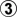, making a one point eye and living easily.

_________________
At some point, doesn't thinking have to go on?

My two main guides in life:
My mother and my wife.Everything with love. Stay safe.

TopDisplay posts from previous: All posts1 day7 days2 weeks1 month3 months6 months1 year Sort by AuthorPost timeSubject AscendingDescending

 All times are UTC - 8 hours [ DST ]

#### Who is online

Users browsing this forum: Majestic-12 [Bot] and 1 guest

 You cannot post new topics in this forumYou cannot reply to topics in this forumYou cannot edit your posts in this forumYou cannot delete your posts in this forumYou cannot post attachments in this forum

Search for:
 Jump to:  Select a forum ------------------ Life In 19x19.com General Topics    Introductions and Guidelines    Off Topic    Announcements    General Go Chat    Beginners    Amateurs    Professionals       Lee Sedol vs Gu Li    Go Rules    Forum/Site Suggestions and Bugs    Creative writing    Tournaments       Ride share to tournaments Improve Your Game    Game Analysis    Study Group    Teachers/Club Leaders       Teacher advertisements    Study Journals L19²GO (Malkovich)    1-on-1 Malkovich games    Big Brother Malkovich games    Rengo Games    Other versions of turn-based games Go Gear    Go Books    Go Book Reviews    Computer Go    Gobans and other equipment    Trading Post    New Products/Upgrades/Sales Go Club Forums    Go Club Discussions       Honinbo Go League    American Go Association Forum       Go Congress 2011 volunteers       AGA volunteers ( non-congress)    Australian Go Association    European Go Federation Forum    Singapore Weiqi Association    KGS    ASR League    IGS    OGS    Tygem    WBaduk    Turn Based Servers    Insei League Events    Kaya.gs       King of the Hill